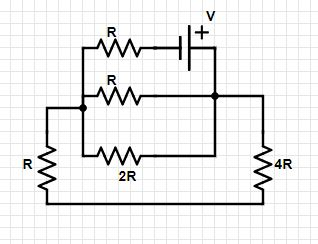# 3. For the circuit shown below. Given [R,V] Calculate. a. The current through the 4R resistor b....

## Question:

3. For the circuit shown below. Given R,V Calculate.

a. The current through the 4R resistor

b. The voltage Vab

c. The equivalent resistance## D.C circuit

D.C circuit is an electrical circuit consisting of D.C voltages and resistances. In a circuit if potential difference across resistors is same then they are in parallel combination and if current passes through resistors one after other than the combination is series.

similarly we have parallel and series combination of cells too.

Effective current or potential difference across any resistor can be found by using kirchoffs laws.

C )

From the figure we see that 4R and R are connected in series and this combination is in parallel to 2R and R

so the effective combination of...

Become a Study.com member to unlock this answer! Create your account